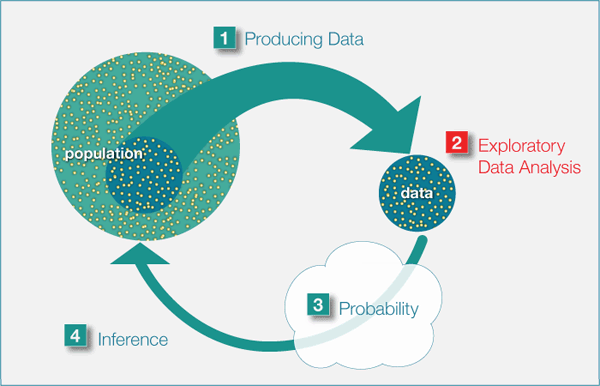## Why It Matters: Nonlinear Models

Before we begin Nonlinear Models, let’s see how the new ideas in this module relate to what we learned in the previous modules, Types of Statistical Studies and Producing Data, Summarizing Data Graphically and Numerically, and Examining Relationships: Quantitative Data.

Recall the Big Picture:

We begin a statistical investigation with a research question. The investigation proceeds with the following steps:

• Produce Data: Determine what to measure, then collect the data. ← Types of Statistical Studies and Producing Data
• Explore the Data: Analyze and summarize the data. Summarizing Data Graphically and Numerically, Examining Relationships: Quantitative Data, Nonlinear Models
• Draw a Conclusion: Use the data, probability and statistical inference to draw a conclusion about the population.

Types of Statistical Studies and Producing Data focused on methods for collecting reliable data. Summarizing Data Graphically and Numerically focused on summarizing and analyzing data for a quantitative variable. Examining Relationships: Quantitative Data focused on linear relationships between two quantitative variables. In Nonlinear Models, we focus on nonlinear relationships between two quantitative variables. In the Big Picture of Statistics, the material in this module is still part of exploratory data analysis.